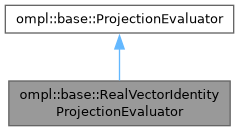ompl::base::RealVectorIdentityProjectionEvaluator Class Reference

Define the identity projection. More...

#include <ompl/base/spaces/RealVectorStateProjections.h>

Inheritance diagram for ompl::base::RealVectorIdentityProjectionEvaluator:[legend]

## Public Member Functions

RealVectorIdentityProjectionEvaluator (const StateSpace *space, const std::vector< double > &cellSizes)
Initialize the identity projection evaluator for state space space. The indices of the kept components are in components and the cell sizes are in cellSizes.

RealVectorIdentityProjectionEvaluator (const StateSpacePtr &space, const std::vector< double > &cellSizes)
Initialize the identity projection evaluator for state space space. The indices of the kept components are in components and the cell sizes are in cellSizes.

RealVectorIdentityProjectionEvaluator (const StateSpace *space)
Initialize the identity projection evaluator for state space space. The indices of the kept components are in components and the cell sizes are a tenth of the bounds from the state space.

RealVectorIdentityProjectionEvaluator (const StateSpacePtr &space)
Initialize the identity projection evaluator for state space space. The indices of the kept components are in components and the cell sizes are a tenth of the bounds from the state space.

unsigned int getDimension () const override
Return the dimension of the projection defined by this evaluator.

void defaultCellSizes () override
Set the default cell dimensions for this projection. The default implementation of this function is empty. setup() calls this function if no cell dimensions have been previously set.

void setup () override
Perform configuration steps, if needed.

void project (const State *state, Eigen::Ref< Eigen::VectorXd > projection) const override
Compute the projection as an array of double values.Public Member Functions inherited from ompl::base::ProjectionEvaluator
ProjectionEvaluator (const ProjectionEvaluator &)=delete

ProjectionEvaluatoroperator= (const ProjectionEvaluator &)=delete

ProjectionEvaluator (const StateSpace *space)
Construct a projection evaluator for a specific state space.

ProjectionEvaluator (const StateSpacePtr &space)
Construct a projection evaluator for a specific state space.

virtual void setCellSizes (const std::vector< double > &cellSizes)
Define the size (in each dimension) of a grid cell. The number of sizes set here must be the same as the dimension of the projection computed by the projection evaluator. After a call to this function, setup() will not call defaultCellSizes() or inferCellSizes() any more.

void setCellSizes (unsigned int dim, double cellSize)
Set the cell sizes to cellSize for a particular dimension dim. This function simply calls getCellSizes(), modifies the desired dimension and then calls setCellSizes(). This is done intentionally to enforce a call to setCellSizes().

void mulCellSizes (double factor)
Multiply the cell sizes in each dimension by a specified factor factor. This function does nothing if cell sizes have not been set. If cell sizes have been set (irrespective of source; e.g., user, default or inferred), this function will then call setCellSizes(), so the source of the cell sizes will be considered to be the user.

bool userConfigured () const
Return true if any user configuration has been done to this projection evaluator (setCellSizes() was called)

const std::vector< double > & getCellSizes () const
Get the size (each dimension) of a grid cell

double getCellSizes (unsigned int dim) const
Get the size of a particular dimension of a grid cell

void checkCellSizes () const
Check if cell dimensions match projection dimension.

void inferCellSizes ()
Sample the state space and decide on default cell sizes. This function is called by setup() if no cell dsizes have been set and defaultCellSizes() does not fill the cell sizes either.

void checkBounds () const
Check if the projection dimension matched the dimension of the bounds.

bool hasBounds () const
Check if bounds were specified for this projection.

void setBounds (const RealVectorBounds &bounds)
Set bounds on the projection. The PDST planner needs to known the bounds on the projection. Default bounds are automatically computed by inferCellSizes().

const RealVectorBoundsgetBounds () const
Get the bounds computed/set for this projection.

void inferBounds ()
Compute an approximation of the bounds for this projection space. getBounds() will then report the computed bounds.

void computeCoordinates (const Eigen::Ref< Eigen::VectorXd > &projection, Eigen::Ref< Eigen::VectorXi > coord) const
Compute integer coordinates for a projection.

void computeCoordinates (const State *state, Eigen::Ref< Eigen::VectorXi > coord) const
Compute integer coordinates for a state.

ParamSetparams ()
Get the parameters for this projection.

const ParamSetparams () const
Get the parameters for this projection.

virtual void printSettings (std::ostream &out=std::cout) const

virtual void printProjection (const Eigen::Ref< Eigen::VectorXd > &projection, std::ostream &out=std::cout) const
Print a euclidean projection.Protected Member Functions inherited from ompl::base::ProjectionEvaluator
void estimateBounds ()
Fill estimatedBounds_ with an approximate bounding box for the projection space (via sampling)Protected Attributes inherited from ompl::base::ProjectionEvaluator
const StateSpacespace_
The state space this projection operates on.

std::vector< double > cellSizes_
The size of a cell, in every dimension of the projected space, in the implicitly defined integer grid.

RealVectorBounds bounds_
A bounding box for projected state values.

RealVectorBounds estimatedBounds_
An approximate bounding box for projected state values; This is the cached result of estimateBounds() which may later be copied to bounds_ if bounds are needed but were not specified.

bool defaultCellSizes_
Flag indicating whether cell sizes have been set by the user, or whether they were inferred automatically. This flag becomes false if setCellSizes() is called.

bool cellSizesWereInferred_
Flag indicating whether projection cell sizes were automatically inferred.

ParamSet params_
The set of parameters for this projection.

## Detailed Description

Define the identity projection.

Definition at line 228 of file RealVectorStateProjections.h.

The documentation for this class was generated from the following files: